Next: 15.3 Convexity Up: 15. The Second Derivative Previous: 15.1 Higher Order Derivatives   Index

# 15.2 Acceleration

15.16   Definition (Acceleration.) If a particle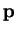moves in a straight line so that its position at time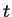is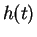, we have defined its velocity at timeto be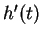. We now define its acceleration at timeto be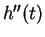, so that acceleration is the derivative of velocity. Thus if a particle moves with a constant acceleration of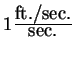, then every second its velocity increases by one ft./sec.

15.17   Example. A mass on the end of a spring moves so that its height at timeis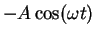, where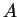and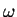are positive numbers. If we denote its velocity at timeby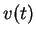, and its acceleration at timeby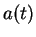then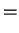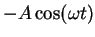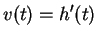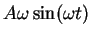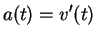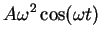From this we see that the acceleration is always of opposite sign from the position: when the mass is above the zero position it is being accelerated downward, and when it is below its equilibrium position it is being accelerated upward. Also we see that the magnitude of the acceleration is largest when the velocity is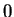.

15.18   Definition (Acceleration due to gravity.) If a particlemoves near the surface of the earth, acted on by no forces except the force due to gravity, thenwill move with a constant acceleration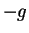which is independent of the mass of. The value of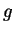is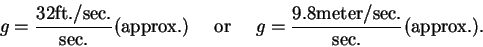We callthe acceleration due to gravity. Actually, the value ofvaries slightly over the surface of the earth, so there is no exact value for. The law just described applies in situations when air resistance and buoyancy can be neglected. It describes the motion of a falling rock well, but it does not describe a falling balloon.

Remark: When I solve applied problems, I will usually omit all units (e.g. feet or seconds) in my calculations, and will put them in only in the final answers.

15.19   Example. A juggler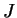tosses a ball vertically upward from a height of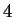feet above the ground with a speed of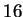ft./sec. Letdenote the height of the ball above the ground at time. We will set our clock so that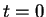corresponds to the time of the toss: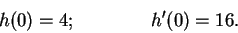We will suppose that while the ball is in the air, its motion is described by a differentiable function of. We assume that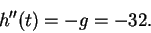We know one function whose derivative is: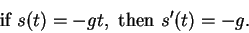By the antiderivative theorem it follows that there is a constant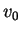such that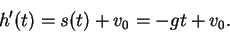Moreover we can calculateas follows: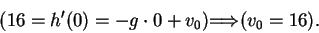Thus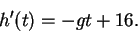We know a function whose derivative is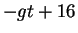: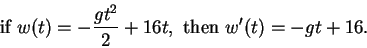Thus there is a constant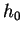such that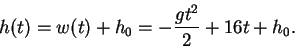To findwe set: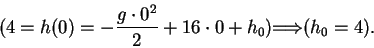Thus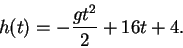The ball will reach its maximum height when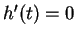, i.e. when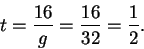The maximum height reached by the ball is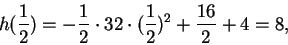so the ball rises to a maximum height of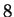feet above the ground.

15.20   Example (Conservation of energy.) Suppose that a particlemoves near the surface of the earth acted upon by no forces except the force of gravity. Letanddenote respectively its height above the earth and its velocity at time. Then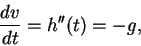so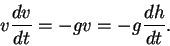Now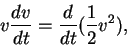so we have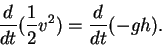It follows that there is a constant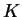such that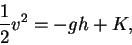or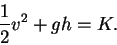If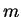is the mass of the particlethen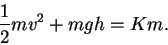(15.21)

The quantity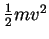is called the kinetic energy of, and the quantity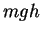is called the potential energy of. Equation (15.21) states that asmoves, the sum of its potential energy end its kinetic energy remains constant.

15.22   Exercise. A A particle moves in a vertical line near the surface of the earth, acted upon by no forces except the force of gravity. At timeit is at height, and has velocity. Derive the formula for the height of the particle at time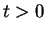.

15.23   Exercise. The acceleration due to gravity on the moon is approximately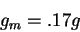wheredenotes the acceleration due to gravity on the earth. A juggleron the moon wants to toss a ball vertically upward so that it rises 4 feet above its starting height. With what velocity should the ball leave's hand?Next: 15.3 Convexity Up: 15. The Second Derivative Previous: 15.1 Higher Order Derivatives   Index
Ray Mayer 2007-09-07